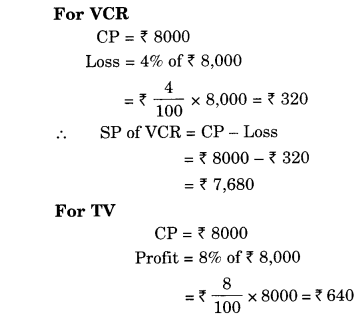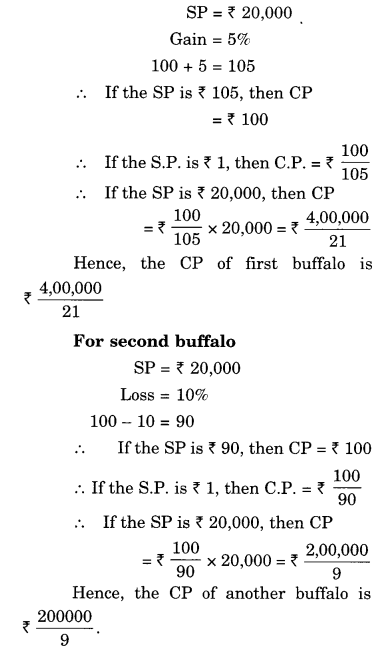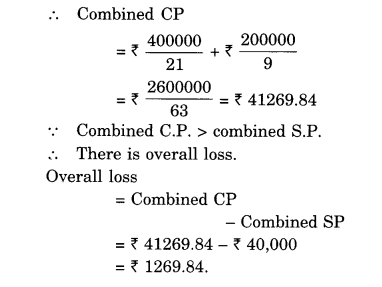NCERT Solutions for Class 8 Maths Chapter 8 Comparing Quantities Ex 8.2 are part of NCERT Solutions for Class 8 Maths. Here we have given NCERT Solutions for Class 8 Maths Chapter 8 Comparing Quantities Ex 8.2.

 Board CBSE Textbook NCERT Class Class 8 Subject Maths Chapter Chapter 8 Chapter Name Comparing Quantities Exercise Ex 8.2 Number of Questions Solved 10 Category NCERT Solutions

## NCERT Solutions for Class 8 Maths Chapter 8 Comparing Quantities Ex 8.2

Question 1.
A man got a 10% increase in his salary. If his new salary is f1,54,000, find his original salary.
Solution.
100 + 10 = 110Question 2.
On Sunday 845 people went to the Zoo. On Monday only 169 people went. What is the percent decrease in the people visiting the Zoo on Monday?
Solution.
Number of people who went to Zoo on Sunday = 845
Number of people who went to Zoo on Monday = 169
∴ Decrease in the number of people who went to Zoo 845 – 169 = 676.
∴Per cent decrease in the number of people visiting the Zoo on Monday
= $$\frac { decrease }{ original\quad number } \times 100%$$
= $$\frac { 676 }{ 845 } \times 100%=80%$$

Question 3.
A shopkeeper buys 80 articles for ₹ 2,400 and sells them for a profit of 16%. Find the selling price of one article.
Solution.
CP of 80 articles = ₹ 2400
Profit = 16% of ₹ 2400
= ₹ $$\frac { 16 }{ 100 } \times 2400$$ = ₹ 384
∴SP of 80 articles
= CP + Profit
= ₹ 2400 + ₹ 384
= ₹ 2784
∴ SP of 1 article = ₹ $$\frac { 2784 }{ 80 }$$ = ₹ 34.80
Hence, the selling price of one article is ₹ 34.80.

Question 4.
The cost of an article was ₹ 15,500, ₹450 were spent on its repairs. If it is sold for a profit of 15%, find the selling price of the article.
Solution.
CP of the article = ₹ 15,500
Money spent on its rapair = ₹ 450
Total CP of the article = ₹ 15,500 + ₹ 450
= ₹ 15,950
Profit = 15% of ₹ 15,950
= ₹ $$\frac { 15 }{ 100 } \times 15,950$$ = ₹ 2392.50
.-. SP of the article
= CP + Profit
=₹ 15,950 + ₹ 2392.50
= ₹ 18342.50
Hence, the selling price of the article is ₹ 18342.50.

Question 5.
A VCR and TV mere bought for ₹ 8,000 each. The shopkeeper made a loss of 4% on the VCR and a profit of 8% on TV. Find the gain or loss percent on the whole transaction.
Solution.
Combined CP of VCR and TV
= ₹ 8,000 + ₹ 8,000 = ₹ 16,000Question 6.
During a sale, a shop offered a discount of 10% on the marked prices of all the items. What would a customer have to pay for a pair of jeans marked at ₹ 1450 and two shirts marked at ₹ 850 each?
Solution.
For a pair of jeans
Marked price = ₹ 1450
Discount rate = 10%
Discount = 10% of ₹ 1450
= ₹ $$\frac { 10 }{ 100 } \times 1450$$ = ₹ 145
∴ Sale price = Marked price – Discount
= ₹ 1450- ₹ 145 = ₹ 1305
For two shirts
Marked price = ₹ 850 x 2 = ₹ 1700
Discount rate = 10%
∴ Discount
= 10% of ₹ 1700
₹ $$\frac { 10 }{ 100 } \times 1700$$ x 1700 = ₹ 170
∴ Sale price
= Marked price – Discount
= ₹ 1700 – ₹ 170 =₹ 1530
= ₹ 1305 + ₹ 1530 = ₹ 2835
Hence, the customer will have to pay ₹ 2835 for a pair of jeans and two shirts.

Question 7.
A milkman sold two of his buffaloes for ₹ 20,000 each. On one he made a gain of 5% and on the other a loss of 10%. Find his overall gain or loss. (Hint: Find CP of each.)
Solution.
Combined SP
= ₹ 20,000 x 2 = ₹ 40,000
For first buffaloQuestion 8.
The price of a TV is ₹ 13,000. The sales tax charged on it is at the rate of 12%. Find the amount that Vinod will have to pay if he buys it.
Solution.
Price of TV = ₹ 13,000
Sales tax charged on it
= 12% of ₹ 13,000
= ₹ $$\frac { 12 }{ 100 } \times 13,000$$ = ₹ 1560
∴ Amount to be paid
= Price + Sales Tax
= ₹ 13,000 + ₹ 1,560
= ₹ 14,560.
Hence, the amount that Vinod will have to pay for the TV if he buys it is ₹ 14,560.

Question 9.
Arun bought a pair of skates at a sale where the discount is given was 20%. If the amount he pays is ₹ 1,600, find the marked price.
Solution.
Amount paid = ₹ 1600
Discount rate = 20%.
100 – 20 = 80
∵ If amount paid is ₹ 80, then the marked price = ₹ 100
∴ If amount paid is ₹ 1, then the marked price = ₹ $$\frac { 100 }{ 80 }$$
∴ If amount paid is ₹ 1600, then the marked price = $$\frac { 100 }{ 80 } \times 1600$$ = ₹ 2000
Hence, the marked price of the pair of skates is ₹ 2000.
Aliter:
Let the market price of the pair of shoes be ₹ 100.
Rate of discount = 20%
∴ Discount = 20% of ₹ 100
= $$\frac { 20 }{ 100 } \times 100$$ = ₹ 20
∴ Sale price = Marked price – Discount
= ₹ 100 – ₹ 20 = ₹ 80
∵ If the sale price is ₹ 80, then, the marked price = ₹ 100
∴ If the sale price is ₹ 1, then the marked price = ₹ $$\frac { 100 }{ 80 }$$
∴ If the sale price is ₹ 1600, then the marked price = ₹ $$\frac { 100 }{ 80 } \times 1600$$ = ₹ 2000
Hence, the marked price of the pair of shoes is ₹ 2000.

Question 10.
I purchased a hair-dryer for ₹ 5,400 including 8% VAT. Find the price before VAT was added.
Solution.
Price of hair-dryer including VAT = ₹ 5400
VAT rate = 8%
100 + 8 = 108
∵ When price including VAT is ₹ 108,
original price = ₹ 100
∴ When price including VAT is ₹ 5,400,
original price = ₹ $$\frac { 100 }{ 108 } \times \left( 5,400 \right)$$ = ₹ 5,000
Hence, the price before VAT was added is ₹ 5,000.

We hope the NCERT Solutions for Class 8 Maths Chapter 8 Comparing Quantities Ex 8.2 help you. If you have any query regarding NCERT Solutions for Class 8 Maths Chapter 8 Comparing Quantities Ex 8.2, drop a comment below and we will get back to you at the earliest.